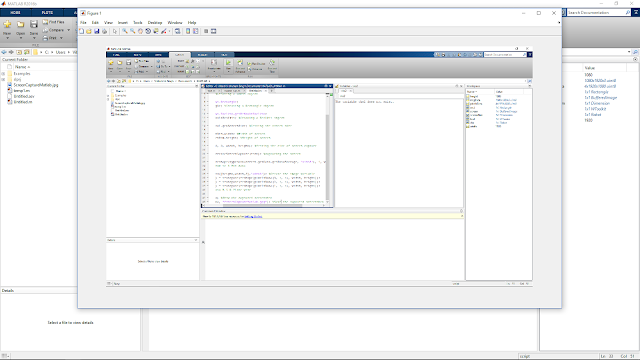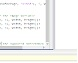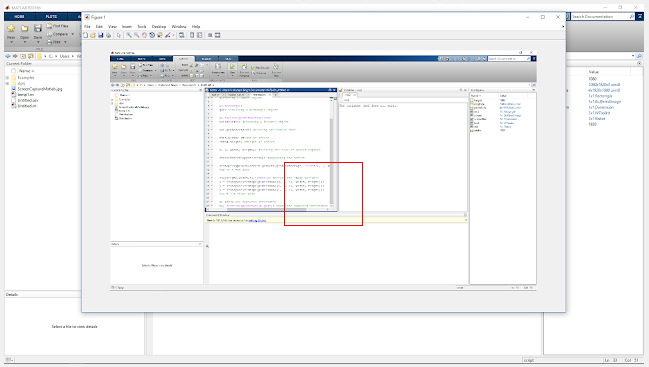Template Matching in Grayscale/RGB image through Normalized Phase Correlation in MATLAB

In image processing, Template Matching is a technique to match a smaller portion of an image with a template image. Or in other words, a technique to find areas in the larger image that match a smaller (template) image.

So why template matching is generally used? For example in a product assembly line, if some of the product after visibly scanning is not matching with a good sample (template of a good sample product) then it could be automatically discarded.  An example is above.

Thus whenever our application needs to match a portion of the image with a reference image to judge its quality/location in a bigger image we need template matching.

Why phase correlation for template matching?
This method is generally fast, accurate, resilient to noise and can withstand the rotation as well as translation of the template within the image!

In our experiment: following is the image in which we will be searching for the template. The following code although tested for a grayscale image but it can work well even for a true-color image as well.Image to match with template (save it as image.jpg for using it in the code)

Template Image:Template image (save it as template.jpg for using it in the code)

MATLAB Code for Template Matching in Grayscale/RGB image through Normalized Phase Correlation
% comment in Bold
% This code provides 2 options to template match i.e., in frequency domain
% through normalized phase correlation. Normxcorr2 is included to act as a benchmark
% for speed and accuracy for both these methods. At the end of the code the
% execution time of processing is also displayed

%% Initial Console/Memory cleaning operations

clc
clear
close all
warning off

%% Options
disp(' Frequency Domain Processing through Normalized Phase Correlation- Can withstand tonal mismatch, translation, occlusion)(Fastest)');
disp(' Normalized Correlation (Matlab Function)- To act as a benchmark for execution time and accuracy ) (Meduium fast)');
option = input('Enter option[1 or 2]:');

%if images in working directory: give just full image name with extension
%if not in the working directory: provide the full path of the image file.

%image which is to be placed on the parent image
% image of whose pattern to be matched on the parent image
% uncomment below if wanted to extract an image portion from the original image to create a new matching template.
% im2match_orig = imcrop(im2process_orig, [100 250 size(im2paste,1)-1 size(im2paste,2)-1]);

if(size(im2process_orig,1)<size(im2match_orig,1)||size(im2process_orig,1)<size(im2match_orig,1))
disp('The size of the template should be smaller than the image! Please try again after correcting this!');
return;
end
%checking for precondition (size of template < size of image)

offset_row=0;
offset_col=0;
%above are the coordinates correction offset introduced by the method

subplot(3,3,[1,2,3,4,5,6])
imshow(im2process_orig); title('Parent image')
hold on
subplot(3,3,7)
imshow(im2match_orig); title('Image pattern to find')
disp('Step 1/5 completed successfully!')
%% Ensuring we get a grayscale image to process

if(size(im2process_orig,3)>1)
im2process    = rgb2gray(im2process_orig);
else
im2process    = im2process_orig;
end
%rgb2gray works as: intensity = 0.2989*red + 0.5870*green + 0.1140*blue
if(size(im2match_orig,3)>1)
im2match    = rgb2gray(im2match_orig);
else
im2match    = im2match_orig;
end

%filtering the template image with an adaptive filter of smallest kernel
%size to smoothen out any noise
%im2match = wiener2(im2match,[2 2]);

%grayscale domain represents luminance and since luminance is by far more
%important in distinguishing visual features we generally process image in
%grayscale domain
disp('Step 2/5 completed successfully!')
tic
%% Constructing a correlation-matrix between images
if option ==2
corr_matrix = normxcorr2(im2match_orig,im2process);
%normalised correlation introduce some offset in the detection point
%that is needed to be corrected, normxcorr2 is a time optimised method
%of mormalized correlation but it is slower that Phase correlation.
offset_row=size(im2match_orig,1);
offset_col=size(im2match_orig,2);
elseif option ==1

fft_parent = fft2((im2process));
%FFT of parent image
fft_match = fft2((im2match), size(im2process,1), size(im2process,2));
%the above statements will automatically pad the template matrix with zeros
%and make it equal in size of image to process. This zero padding is
%also require to reduce the edge effects
corr_matrix = real(ifft2(((fft_parent.*conj(fft_match))./abs(fft_parent.*conj(fft_match)))));
%corr_matrix contains the ifft of normalised cross correlation of
%template and image of whose the maximum value will give the coordinates
%of matched template

else
disp('Wrong Choice! Try Again!');
return;
end
disp('Step 3/5 completed successfully!')
%finding the maximum value of correlation coefficient and its index
n_peak=10;
peak_sum=zeros(1,n_peak);
row=zeros(1,n_peak);
col=zeros(1,n_peak);

[~,I] = max(corr_matrix(:));
[row(1), col(1)] = ind2sub(size(corr_matrix),I);
if ((row(1)>1 && row(1)<size(im2process,1)) && (col(1)>1 && col(1)<size(im2process,2)))
peak_sum(1)=mean(corr_matrix(row(1), col(1)) + corr_matrix(row(1)-1, col(1)-1) + corr_matrix(row(1)-1, col(1)) + corr_matrix(row(1)-1, col(1)+1) + ...
corr_matrix(row(1), col(1)-1) + corr_matrix(row(1), col(1)) + corr_matrix(row(1), col(1)+1) + ...
corr_matrix(row(1)+1, col(1)-1) + corr_matrix(row(1)+1, col(1)) + corr_matrix(row(1)+1, col(1)+1));
end
%this averaging will ensure that the detected peak is a desired detection
%works on a logic that due to noise we could get an abrupt peak in cross
%correlation matrix but the neighbouring points around the peak will also
%have weights which when averaged would be higher for the actual match

for l=2:n_peak
[~,I] = max(corr_matrix(:));
[row(l), col(l)] = ind2sub(size(corr_matrix),I);

if ((row(l)>1 && row(l)<size(im2process,1)) && (col(l)>1 && col(l)<size(im2process,2)))

peak_sum(l)=mean(corr_matrix(row(l), col(l)) + corr_matrix(row(l)-1, col(l)-1) + corr_matrix(row(l)-1, col(l)) + corr_matrix(row(l)-1, col(l)+1) + ...
corr_matrix(row(l), col(l)-1) + corr_matrix(row(l), col(l)) + corr_matrix(row(l), col(l)+1) + ...
corr_matrix(row(l)+1, col(l)-1) + corr_matrix(row(l)+1, col(l)) + corr_matrix(row(l)+1, col(l)+1));
corr_matrix(row(l), col(l))=0;

end
%this averaging will ensure that the detected peak is a desired detection
%works on a logic that due to noise we could get an abrupt peak in cross
%correlation matrix but the neighboring points around the peak will also
%have weights which when averaged would be higher for the actual match
end
[~,max_corrval]=max(peak_sum(:));

row_f=row(max_corrval)-offset_row;
col_f=col(max_corrval)-offset_col;
%% Display the pattern matching result on parent/original image

figure;
imshow(im2process_orig); hold on;
rectangle('Position', [col_f row_f size(im2match,2) size(im2match,1)], 'EdgeColor', [1 0 0]);
title('Pattern matching result (red rectangle)')
disp('Step 4/5 completed successfully!')
%% Display the processed image-after replacing the matched portion

disp('Step 5/5 completed successfully!')
toc

%toc will display the elapsed time of the also to proceed.Result of above pattern matching using normalised phase correlation.

The result of template matching with above code for normalized phase correlation:

Have you got a great idea with this code or with template matching in MATLAB? You can share with in the comments!

Author: Vibhutesh Kumar SinghAn active & prominent author at Digital iVision Labs! Like to write about MATLAB, C++, Arduino, OpenCV, NI Labview, Web Designing & other Electronics stuffs! Finished up M.Tech. From IIIT Delhi, now doing PhD in wireless communication with prominent researchers University College Dublin (CONNECT CENTRE). Drop a mail: vibhutesh[at]gmail.com to ask something about the article or Follow him at....